Miscellaneous

Chapter 8 Class 12 Application of Integrals
Serial order wise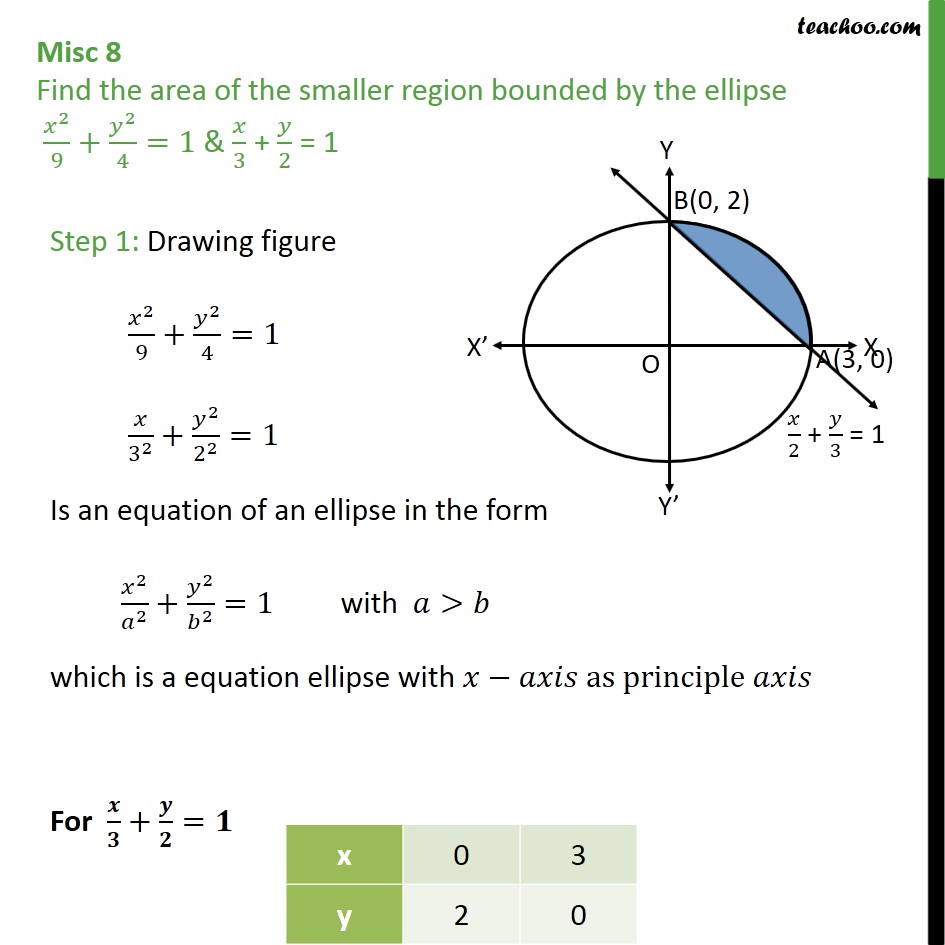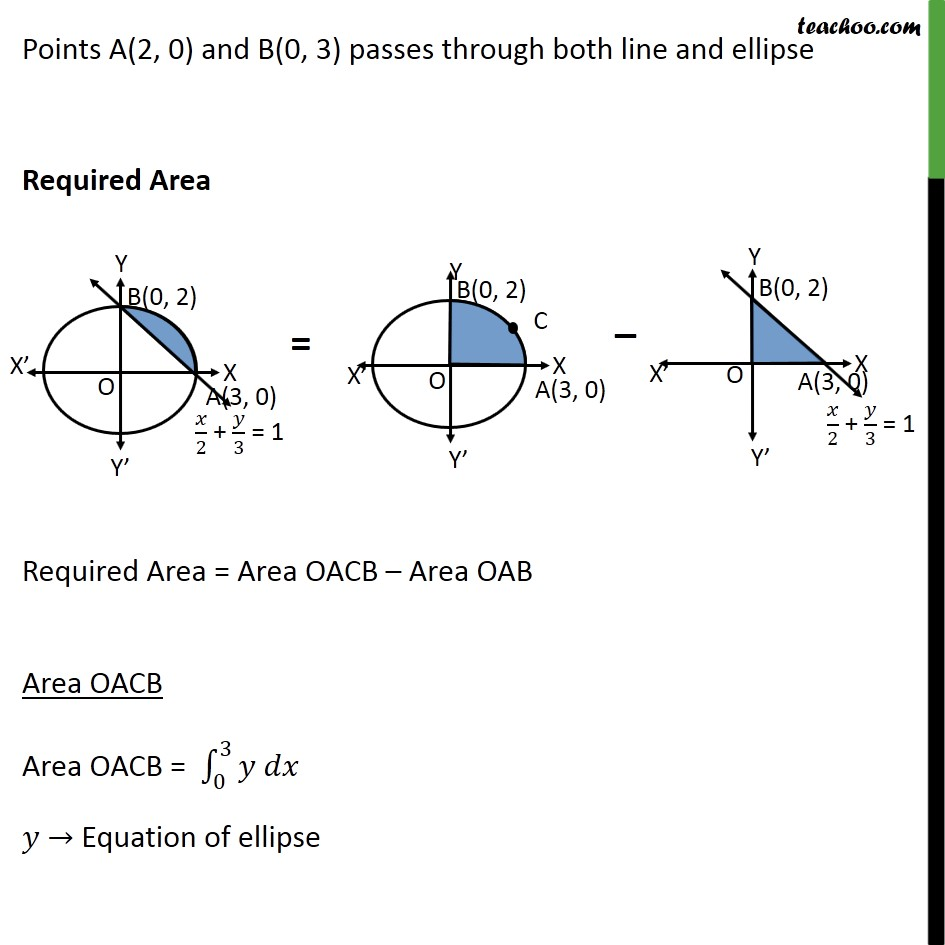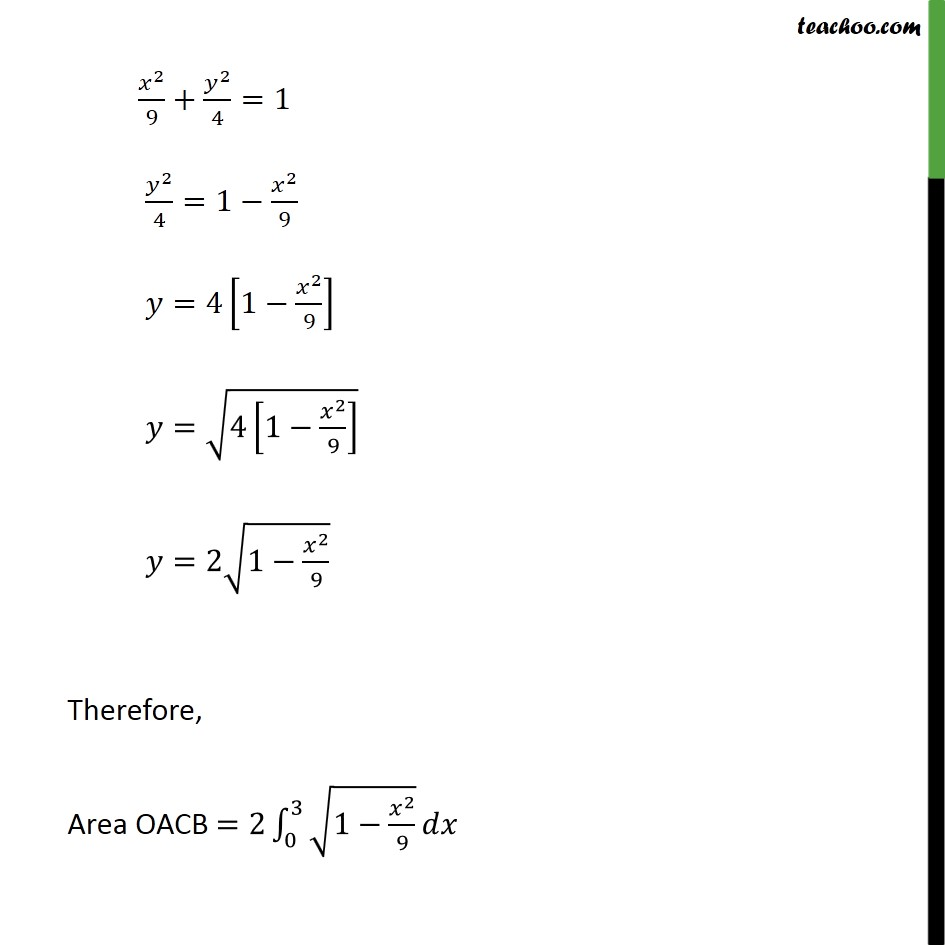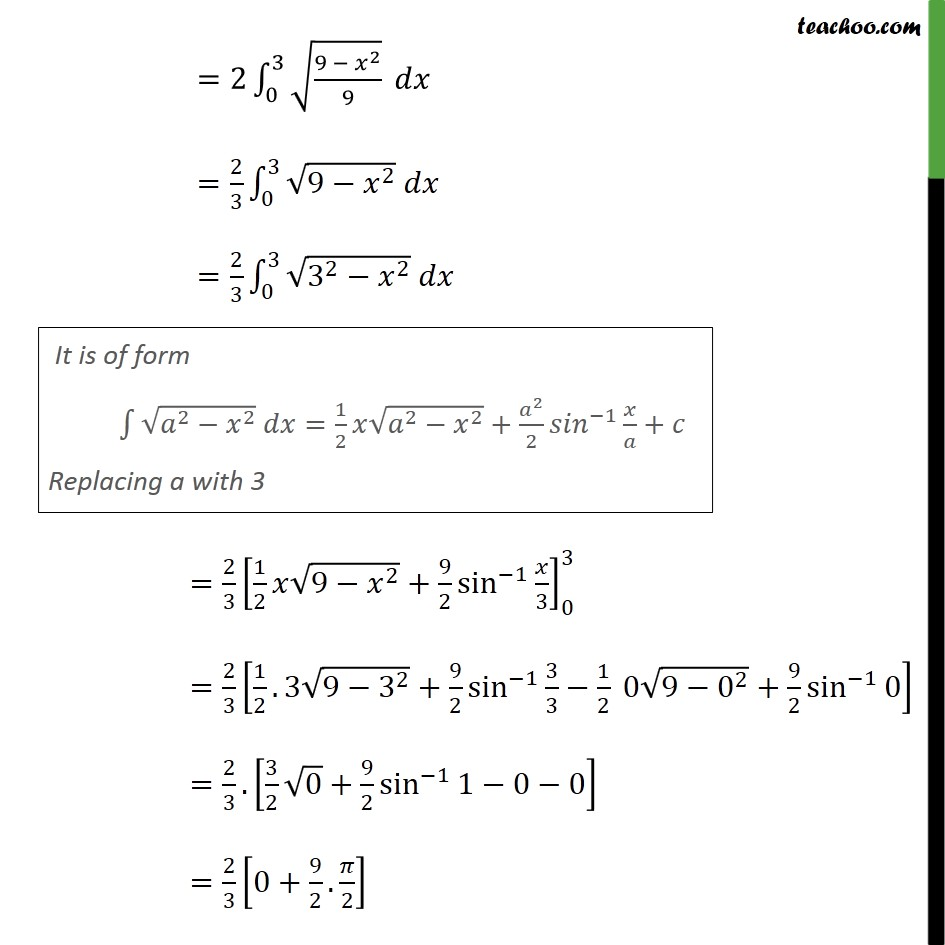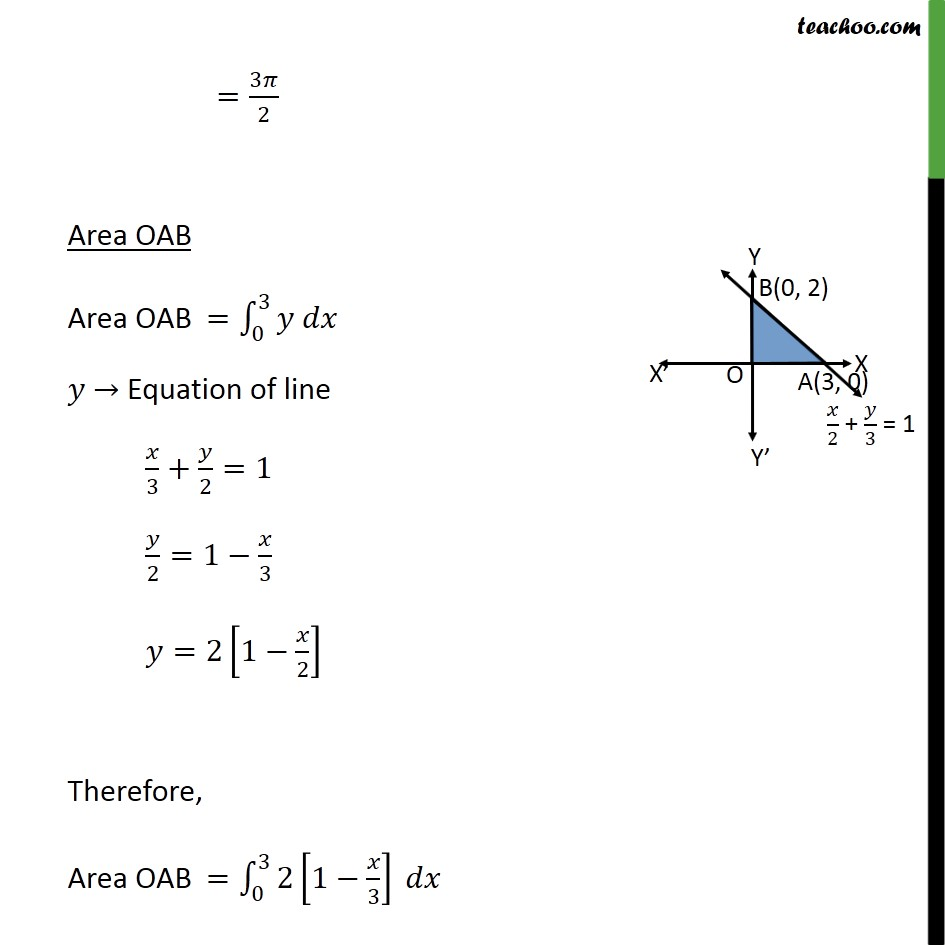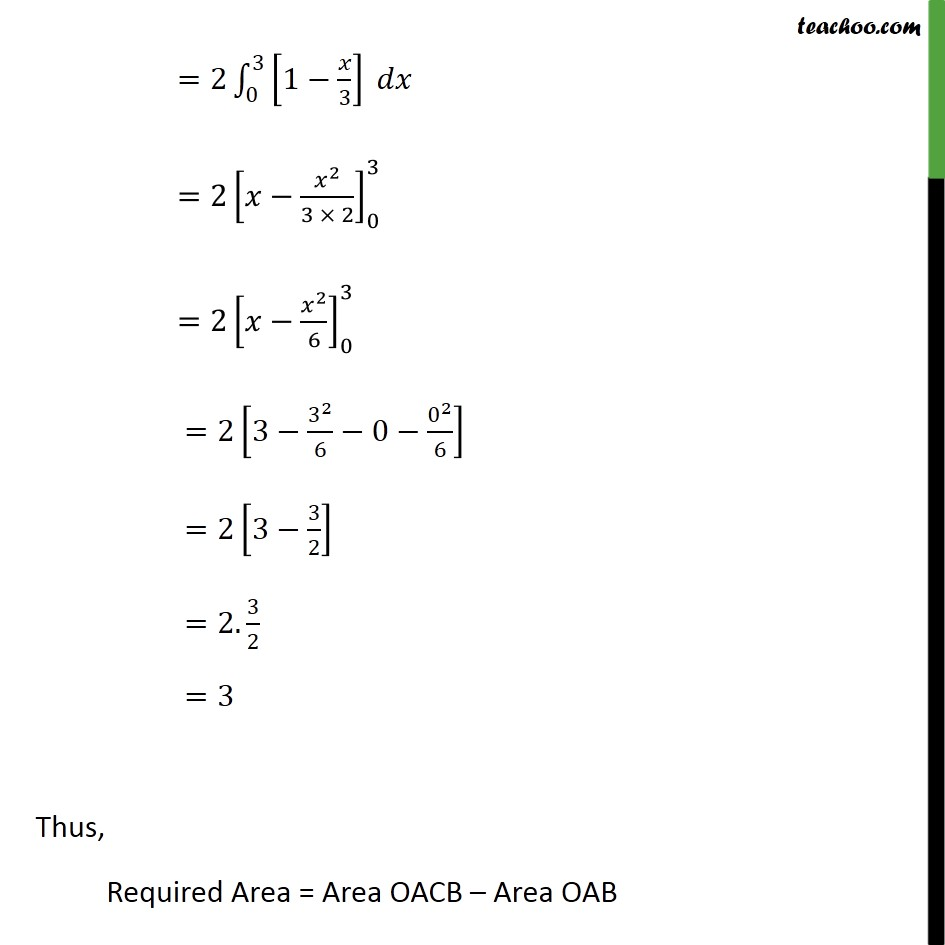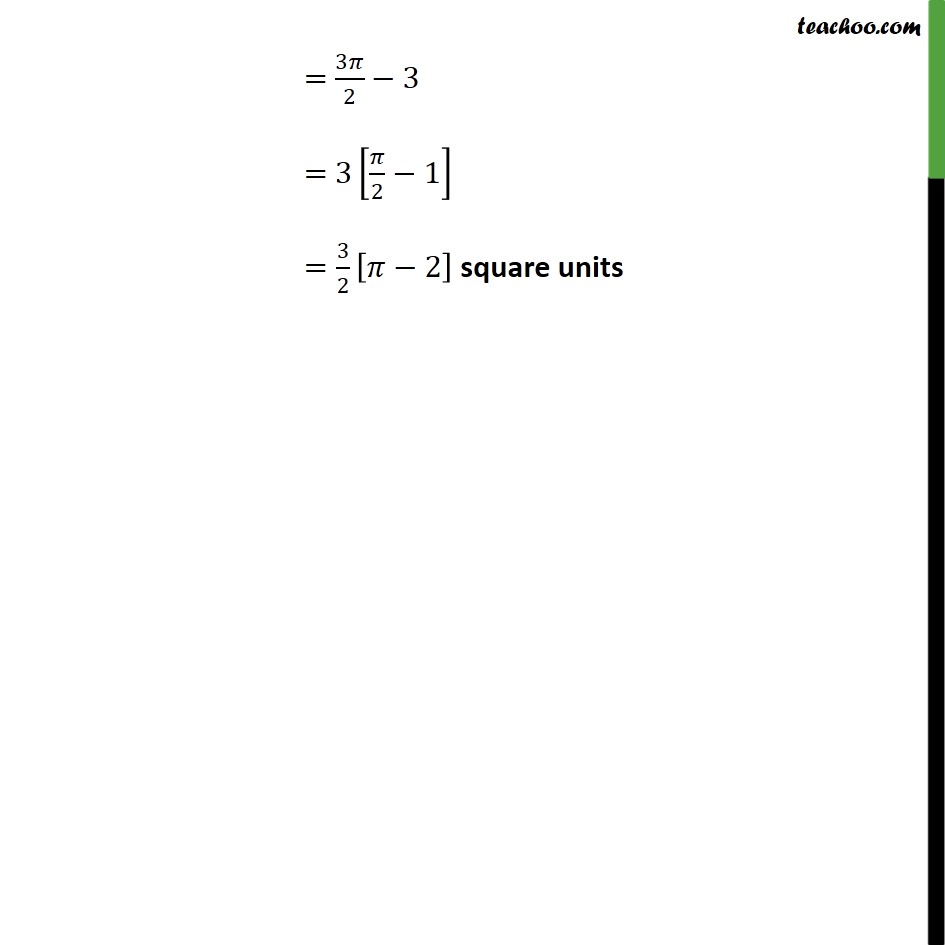Learn in your speed, with individual attention - Teachoo Maths 1-on-1 Class

### Transcript

Question 5 Find the area of the smaller region bounded by the ellipse 2 9 + 2 4 =1 & 3 + 2 = 1 Step 1: Drawing figure 2 9 + 2 4 =1 3 2 + 2 2 2 =1 Is an equation of an ellipse in the form 2 2 + 2 2 =1 with > which is a equation ellipse with as principle For + = Points A(2, 0) and B(0, 3) passes through both line and ellipse Required Area Required Area = Area OACB Area OAB Area OACB Area OACB = 0 3 Equation of ellipse 2 9 + 2 4 =1 2 4 =1 2 9 =4 1 2 9 = 4 1 2 9 =2 1 2 9 Therefore, Area OACB =2 0 3 1 2 9 =2 0 3 9 2 9 = 2 3 0 3 9 2 = 2 3 0 3 3 2 2 = 2 3 1 2 9 2 + 9 2 sin 1 3 0 3 = 2 3 1 2 .3 9 3 2 + 9 2 sin 1 3 3 1 2 0 9 0 2 + 9 2 sin 1 0 = 2 3 . 3 2 0 + 9 2 sin 1 1 0 0 = 2 3 0+ 9 2 . 2 = 3 2 Area OAB Area OAB = 0 3 Equation of line 3 + 2 =1 2 =1 3 =2 1 2 Therefore, Area OAB = 0 3 2 1 3 =2 0 3 1 3 =2 2 3 2 0 3 =2 2 6 0 3 =2 3 3 2 6 0 0 2 6 =2 3 3 2 =2. 3 2 =3 Thus, Required Area = Area OACB Area OAB = 3 2 3 = 3 2 1 = 3 2 2 square units